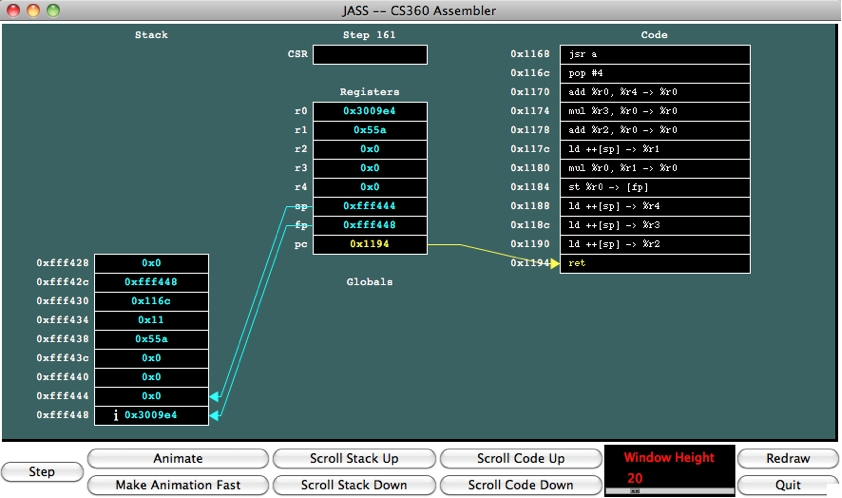## CS360 Lecture notes -- Assembler Lecture #2: Procedures, Stack Frames, Spilling

This lecture is a continuation of computer organization & stack frames. The focus is on procedure calls.

Look at the following C code (p1.c):

 ```int a() { return 1; } int main() { int i; i = a(); } ```

This compiles into assembler that looks like the following:

```    a:
mov #1 -> %r0
ret
main:
push #4
jsr a
st %r0 -> [fp]
ret
```
Both of these procedure calls are straightforward. Main() first allocates one variable on the stack, and then calls "jsr a", which means jump to subroutine a. All a() does is return 1 to its caller -- it does that by setting r0 to one, and then calling "ret". When control returns to main() it stores a's return value, which is in r0, to the memory that it has allocated for i. And it returns.

This seems simple; however, what goes on when jsr and ret are called is a little trickier. This is what happens:

When "jsr" is called, (pc+4) and the current value of fp are both pushed onto the stack. Because of the pushing, the sp's value will be 8 less than it was before the jsr call. Then, the fp is changed to be the current sp, and pc is changed to be the location of the first instruction of the named procedure. This is done atomically by the computer's hardware. After jsr has taken effect, we are in a new stack frame, and the pc is executing a().

When "ret" is called, the sp is changed to be the current fp. Then the fp is popped off the stack: The sp's value is incremented by four, and the fp is read from the stack. Finally, the pc is popped off the stack: The sp's value is incremented by four again, and the pc is read from the stack. Like "jsr", this is all done atomically by the hardware. When "ret" completes, the pc is set to be the instruction after the original "jsr" instruction, and the stack frame of that procedure has been restored.

Let's look at it pictorally. Below is a drawing of what you'll see if you run jassem.tcl on p1.jas. At the start of the program, the stack and registers look as follows: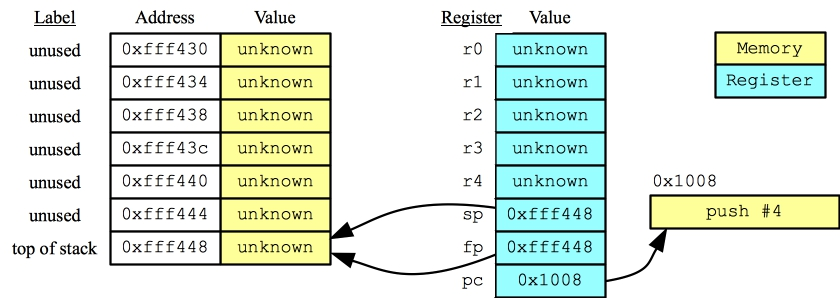(As an aside -- jassem.tcl assigns zero to unknown values and registers. Here, I'm putting "unknown" to show that we don't know what the values will really be.)

First, the sp is decremented by 4 to allocate the local variable i:Now jsr is called. This pushes (pc+4) and the value of the fp on the stack, and sets the fp to the new sp, and pc to a: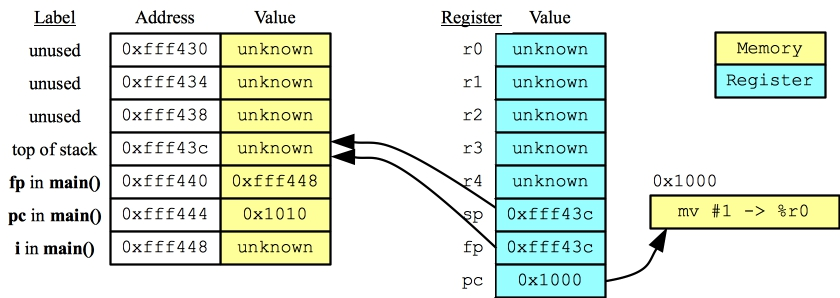Note now that we have a new stack frame for a, and the pc is executing a. The first thing it does is load 1 into r0: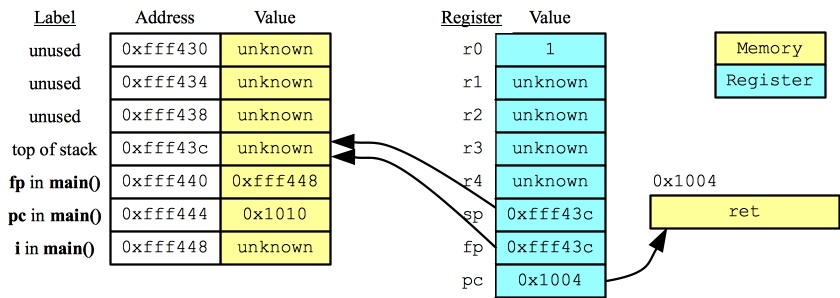We then call "ret". "Ret" sets the sp to the fp (which involves nothing in this case), and then pops the fp and the pc off the stack. When it's done we're back to main()'s stack frame, and executing the next instruction after the jsr: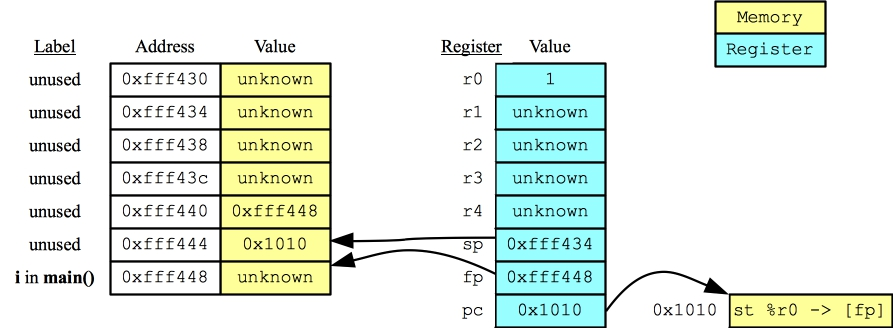Note the "fp in main" and "pc in main" values don't get changed or erased. They simply remain on the stack. However since they are "above the stack", they should not get referenced. Now the "st %r0 -> [fp]" gets executed, and the machine state looks like: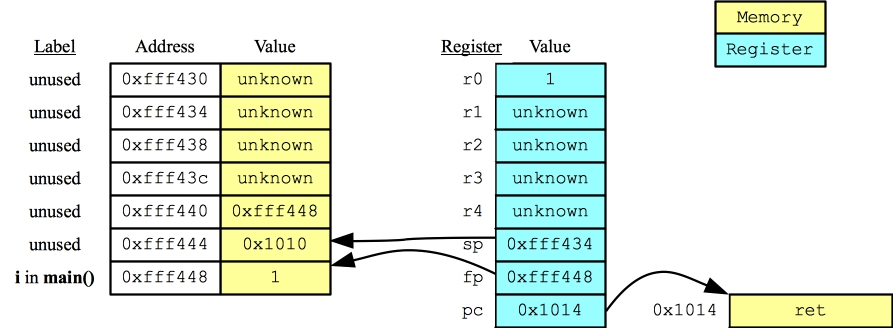Now main() is over, and calls "ret". You can imagine what this does -- the stack is set up so that when main() calls ret, control returns to the operating system and the process goes away.

Now, make sure you go over this with Jassem. The program is in p1.jas, and you should see exactly what I have shown above.

The next example shows a procedure with arguments and local variables. It's a pretty meaty example. The C code (p2.c): is to the left, and the assembler (p2.jas): is to the right:

 ```int a(int i, int j) { int k; i++; j -= 2; k = i * j; return k; } int main() { int i, j, k; i = 3; j = 4; k = a(j+1, i); return 0; } ``` ```a: push #4 / Allocate k, which will be [fp] ld [fp+12] -> %r0 / i++ add %r0, %g1 -> %r0 st %r0 -> [fp+12] ld [fp+16] -> %r0 / j -= 2 mov #2 -> %r1 sub %r0, %r1 -> %r0 st %r0 -> [fp+16] ld [fp+12] -> %r0 / k = i * j ld [fp+16] -> %r1 mul %r0, %r1 -> %r0 st %r0 -> [fp] ld [fp] -> %r0 / return k ret main: push #12 / Allocate i, j, k. / i is [fp-8], j is [fp-4], k is [fp] mov #3 -> %r0 / i = 3 st %r0 -> [fp-8] mov #4 -> %r0 / j = 4 st %r0 -> [fp-4] ld [fp-8] -> %r0 / Push i onto the stack st %r0 -> [sp]-- ld [fp-4] -> %r0 / Push j+1 onto the stack add %r0, %g1 -> %r0 st %r0 -> [sp]-- jsr a / Call a(), then pop the arguments pop #8 st %r0 -> [fp] / Put the return value into k mov #0 -> %r0 / Return 0 ret ```

Let's focus first on the main(). In the beginning, it decrements the stack pointer by 12, which allocates, i, j and k on the stack. Our compiler puts them in the order in which they are declared on the stack, so as the comments above state: i is at [fp-8], j is at [fp-4] and k is at [fp]. Next, we initialize i and j. That is straightforward.

The procedure call requires explanation. When you call a procedure with arguments, what you do is push the arguments onto the stack in reverse order. Then you call jsr. When the jsr call returns, you pop the arguments off the stack, so that you can reuse that memory.

In this case, let's take a look at the stack when main() calls jsr. I'm going to draw this myself, but the values and addresses match up with what happens when you run this in jassem: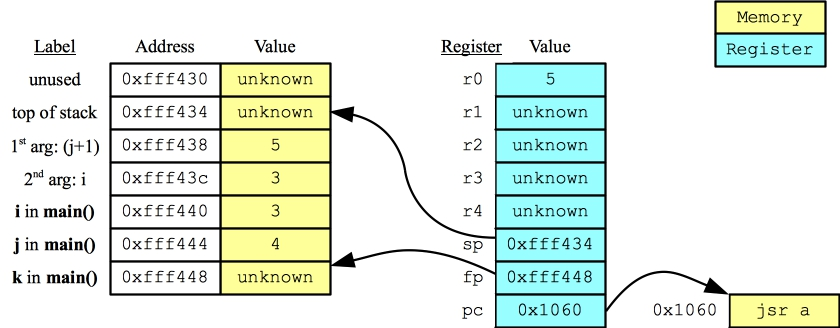It's good practice to label the stack -- you should be able to account for every word.

Now, the jsr statement pushes (pc+4) onto the stack, and then the value of the fp. It then sets the pc to the first instruction in a. That instruction is push #4, so that it allocates k. At this point, here are the labeled stack values and registers. You'll note, I've relabeled the two arguments that were pushed onto the stack as "i in a()" and "j in a()":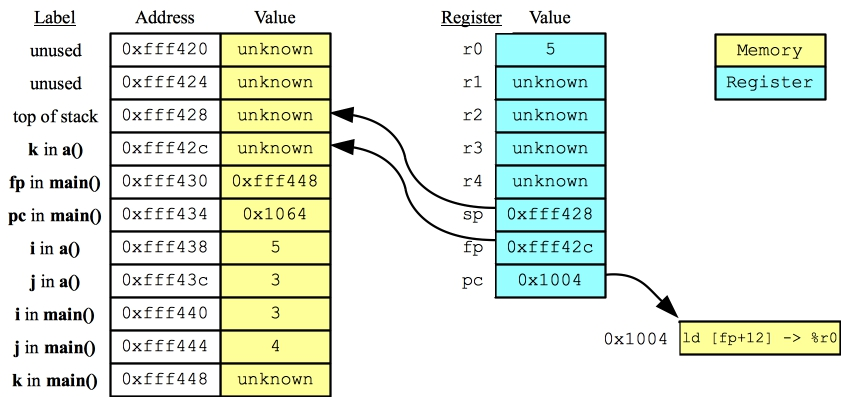Now, let's consider how a() finds its two arguments. It knows that the old fp is in [fp+4] and the old pc is in [fp+8]. Since the arguments are pushed in reverse order, the first argument should be next, at [fp+12]. The second argument is at [fp+16]. If there were a third argument, it would be at [fp+20], and so on.

Now, let's look at the state when a() returns. At that point, you should see that a()'s variables i, j and k are equal to 6, 1 and 6 respectively. k's value has been loaded into the register r0, as that is how return values are passed from one procedure to another: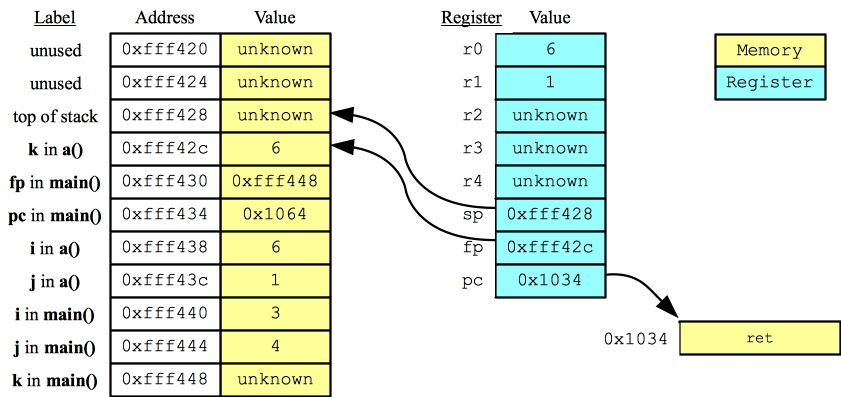Finally, when the pc is set to 0x1064 and the fp is set back to 0xfff448, we are back in main(). The stack pointer is popped eight bytes, and the return value is stored into k. When main() returns, the system looks as follows: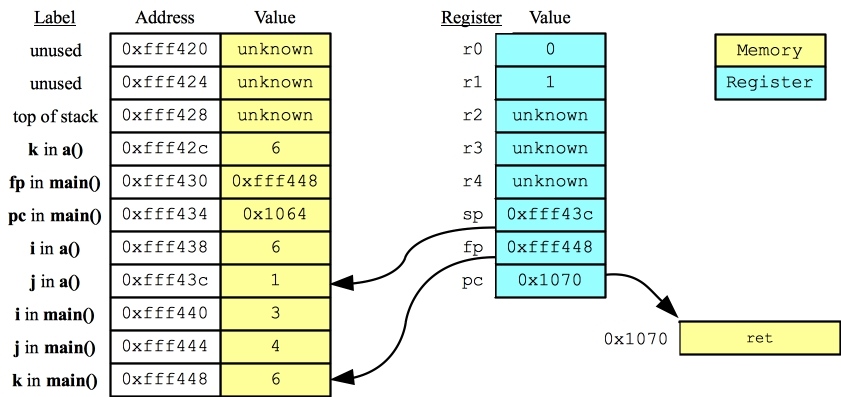Let's go over all of the main points from this example (including some review):

• The jsr statement does four things: It pushes (pc+4) onto the stack, then fp, then it sets the fp to the current value of the sp, and the pc to the first instruction of the given procedure.

• The ret statement undoes what jsr has set up: It sets the sp to the current value of the fp, then it pops the fp and the pc off of the stack.

• If a procedure has arguments, you need to push them onto the stack in reverse order, before making the jsr call.

• Thus, when a procedure is called, its first argument is at [fp+12]. Its second argument is at [fp+16]. Its third argument is at [fp+20]. And so on.

• When a procedure returns a value to its caller, it stores the value in r0.

• If you have pushed arguments before calling jsr, then the very first thing that you should do when the jsr call returns is pop those arguments off the stack.

• Although you are not currently using values whose addresses are less than or equal to the stack pointer, those addresses will have values, and those values may be deterministic. For example, in that last picture, there are a lot of values above the stack pointer, and we know what all of them are between 0xfff42c and 0xfff43c, because we know what the previous instructions have done.

• You should be able to see how each procedure gets its own memory locations for its parameters and local variables. Even though main() and a() each used i, j and k, they had their own separate memory locations for them.

• You should also see how memory gets allocated for local variables and parameters when a procedure executes, and how it goes away when the procedure is done.

As always, I advocate running jassem.tcl on this program yourself and making sure you understand what's going on.

## Register Spilling

One important thing that has to be decided is whether a procedure may use a register without worrying about its current value (like a() does with r0), or whether a procedure should first save the register on the stack before using it. This matters, because suppose for example, that the main routine uses register r3, then calls "jsr a", and afterwards expects r3 to have the same value. Then a() and any procedures that a() calls must make sure not to use r3, or to save r3's value before using it, and restore it when its done.

The act of saving a register's value before the body of a procedure call and restoring it afterwards is called spilling. Different machines and compilers handle spilling in different ways. For example, older CISC architectures sometimes had a spill-mask that would be part of a procedure call. This specified which registers should be spilled, and the machine actually did the spilling for you.

What we do on our machine is a typical spilling solution: Procedures can use r0 and r1 without worrying about their values. However, registers r2 through r4 must be spilled if a procedure uses them.

Here's an example (spill1.c):

 ```int a(int i, int j) { int k; k = (i+2)*(j-5); return k; } int main() { int i; i = a(44, 22); } ```

To compile arithmetic expressions into assembler, it's useful to turn them into trees. For example, the above expression becomes: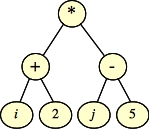In order to evaluate the tree, you need to do a postorder traversal (or, if you think of the edges are pointing upward, you need to do a topological sorting of the tree). Arithmetic has to be done on a register-by-register basis, so each of those nodes must be in a register. You (the compiler) must figure out an ordering of instructions that is legal, and then an assignment of nodes to registers so that you don't reuse registers unless you can be sure that you don't need their values any more.

For example, in the above expression, suppose you do the (i+2) calculation first and hold the result in r0. Then you can't use r0 to calculate (j-5). For that reason, you are going to have to use r2, and because you are using r2, you'll have to spill it onto the stack. I do this at the beginning of a procedure. Then at the end, I "unspill" it by reading it back from the stack.

The code is in spill1.jas, which I've reproduced below. You may use jassem.tcl to step through this.

 ```a: push #4 / Allocate k st %r2 -> [sp]-- / Spill r2 ld [fp+12] -> %r0 mov #2 -> %r1 add %r0, %r1 -> %r0 / Calculate (i+2) and put the result in r0 ld [fp+16] -> %r1 mov #5 -> %r2 sub %r1, %r2 -> %r1 / Calculate (j-5) and put the result in r1 mul %r0, %r1 -> %r0 st %r0 -> [fp] / Do k = r0 * r1 ld [fp] -> %r0 ld ++[sp] -> %r2 / Unspill r2 ret main: push #4 / Allocate i mov #22 -> %r0 / Push arguments onto the stack in reverse order st %r0 -> [sp]-- mov #44 -> %r0 st %r0 -> [sp]-- jsr a pop #8 / Always pop the arguments off the stack after jsr st %r0 -> [fp] ret ```

Note, that you have to spill r2 onto the stack after allocating the local variable. Otherwise, k will not be at [fp]. Think about it.

## An example to show why spilling matters

Take a look at spill2.c:

 ```int a(int i, int j) { int k; k = (i+2)*(j-5); return k; } int main() { int i; i = (a(10, 20) + a(30, 40)); } ```

You'll note that a() is exactly the same. The only difference is that we're calling a() twice, and adding up the return values. Think about that for a minute -- where should you store the return value of the first call to a()? You can't store it in r0 or r1 because making a procedure call will destroy them (we have to assume that). Therefore, you have to store it in a higher register, like r2. You know that's ok, because a() will make sure that r2's value is unchanged. Here's the code for main() (in spill2.jas). You'll notice that main() spills r2 as well, because if any procedure uses r2, r3 or r4, it must spill them.

 ```main: push #4 / Allocate i st %r2 -> [sp]-- / Spill r2 mov #20 -> %r0 / Call a(10, 20) and store the result in r2 st %r0 -> [sp]-- mov #10 -> %r0 st %r0 -> [sp]-- jsr a pop #8 mov %r0 -> %r2 mov #40 -> %r0 / Call a(30, 40) and add the result to r2 st %r0 -> [sp]-- mov #30 -> %r0 st %r0 -> [sp]-- jsr a pop #8 add %r0, %r2 -> %r0 st %r0 -> [fp] ld ++[sp] -> %r2 / Unspill r2 ret ```

Once again, I urge you to trace through this code with jassem to see how the spilling works.

## What do you do when you run out of registers?

Try spill3.c on for size:

 ```int a(int i) { return i+5; } int main() { int i; i = ( (a(2)+a(3)) * (a(4)+a(5)) + (a(10)+a(11)) * (a(12)+a(13)) ) * ( (a(6)+a(7)) * (a(8)+a(9)) + (a(14)+a(15)) * (a(16)+a(17)) ); } ```

Yuck. Here's the expression tree: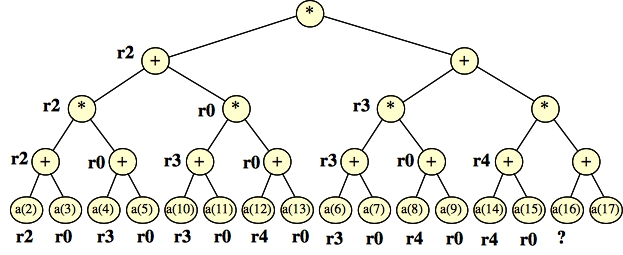You can see I've labeled it with the registers that you can use if you do the calculation in post-order, from left to right. You'll see that we've run out of registers!

Below, I show how you handle that -- you spill the intermediate value shown as "Spill". That allows you to use r2 again, and you no longer run out of registers. Before you do the last multiplication, you unspill the value into a register: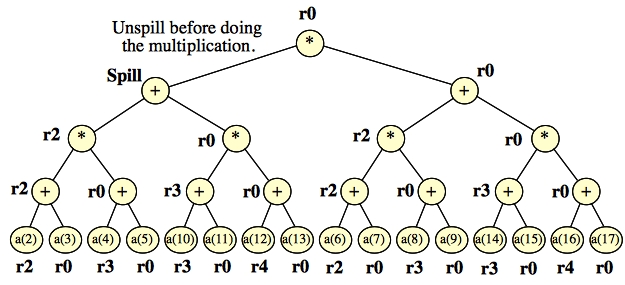Did you really want to see the assembler for that? It's in spill3.jas. It's not that hard to read. Here's the crucial code: Spilling the result of the sum (by the "Spill" in the picture above) onto the stack. I start with the call to a(13). When it's done, you perform the multiplication and addition, and then spill the result of the addition onto the stack. Then you start working on the right side of the equation (starting with a(6)):

 ``` ... mov #13 -> %r0 st %r0 -> [sp]-- jsr a pop #4 add %r0, %r4 -> %r0 mul %r3, %r0 -> %r0 / Multiplication, then Addition, then spill add %r2, %r0 -> %r0 st %r0 -> [sp]-- mov #6 -> %r0 / a(6)+a(7) st %r0 -> [sp]-- jsr a pop #4 mov %r0 -> %r2 ... ```

At the end, when you're done with a(17), you do the multiplication and addition. You have one more multiplication, but its operand is the one spilled to the stack. You unspill it and perform the multiplication. Then you're done!

 ``` ... mov #17 -> %r0 st %r0 -> [sp]-- jsr a pop #4 add %r0, %r4 -> %r0 mul %r3, %r0 -> %r0 / Multiplication, then addition, then unspill and multiply add %r2, %r0 -> %r0 ld ++[sp] -> %r1 mul %r0, %r1 -> %r0 st %r0 -> [fp] / Store the result into i ld ++[sp] -> %r4 / Unspill before returning ld ++[sp] -> %r3 ld ++[sp] -> %r2 ret ```

You can run jassem on this -- it's a bit cumbersome, but I have important screen shots. Here is the state just before the crucial spill: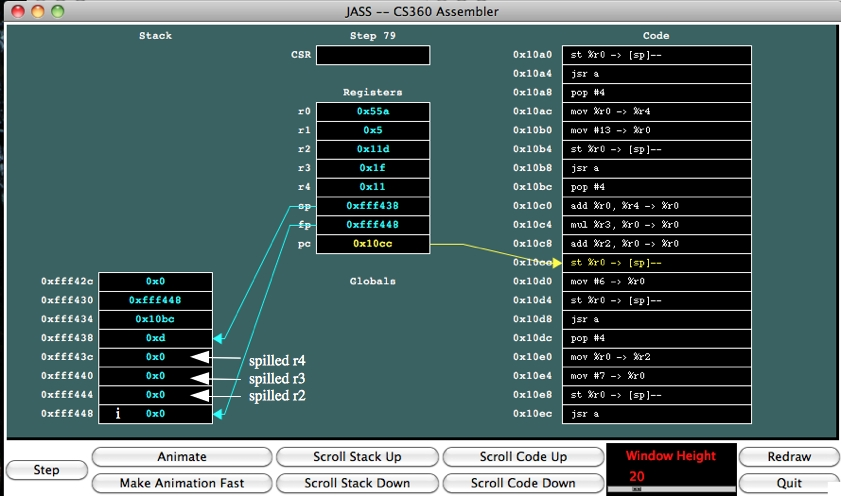You can double-check yourself -- a(i) simply adds 5 to i, so:

• r4 should equal (12+5) = 17 = 0x11.
• r3 should equal (10+5+11+5) = 31 = 0x1f.
• r2 should equal (2+5+3+5)*(4+5+5+5) = 15*19 = 285 = 0x11d
After the spill, 0x55a goes onto the stack: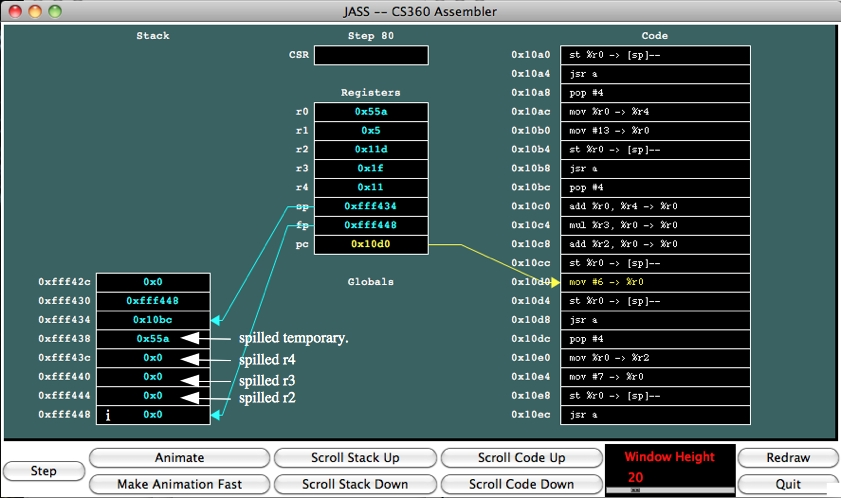I continue stepping to the "unspill":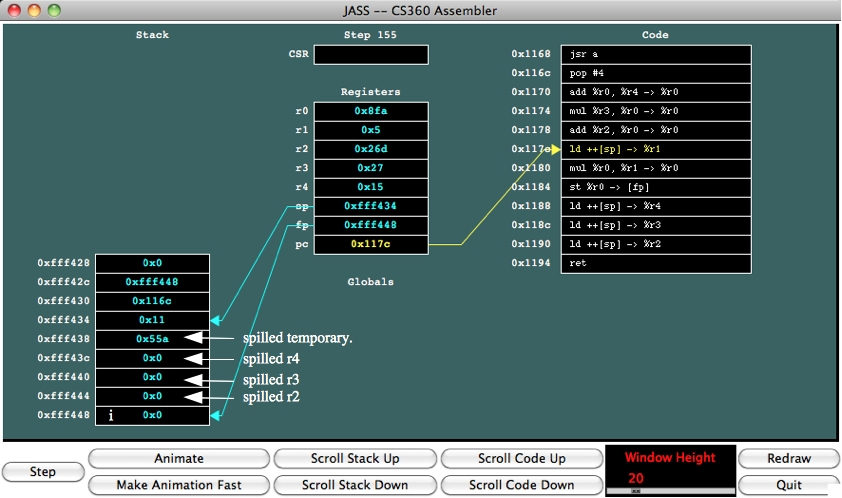And at the end, i has been set to 0x3009e4 = 3148620. Is that right? I'll let you double-check it yourself, but it is indeed correct!